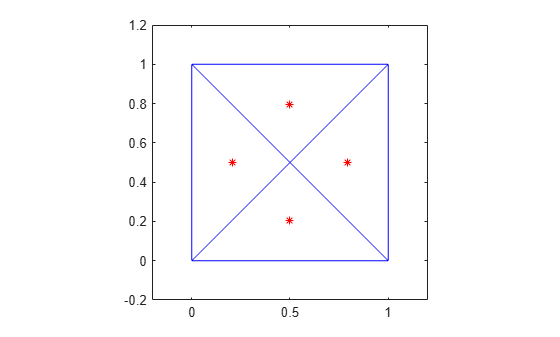incenter

Incenter of triangulation elements

Description

example

C = incenter(TR) returns the coordinates of the incenters of each triangle or tetrahedron in the triangulation TR.

example

C = incenter(TR,ID) returns the coordinates of the incenter of each triangle or tetrahedron specified by ID. The identification numbers of the triangles or tetrahedra in TR are the corresponding row numbers of the property TR.ConnectivityList.

example

[C,r] = incenter(___) also returns the radii of the inscribed circles or spheres.

Examples

collapse all

Create a 2-D Delaunay triangulation.

x = [0 1 1 0 0.5]';
y = [0 0 1 1 0.5]';
TR = delaunayTriangulation(x,y);

Compute the incenters of the triangles.

C = incenter(TR);

Plot the triangles and incenters.

triplot(TR)
axis equal
axis([-0.2 1.2 -0.2 1.2])
hold on
plot(C(:,1),C(:,2),'*r')Calculate the incenter coordinates of the first five tetrahedra in the triangulation, in addition to the radii of their inscribed spheres.

TR = triangulation(tet,X);
[C,r] = incenter(TR,[1:5]')
C = 5×3

-6.1083  -31.0234    8.1439
-2.1439  -31.0283    5.8742
-1.9555  -31.9463    7.4112
-4.3019  -30.8460   10.5169
-3.1596  -29.3642    6.1851

r = 5×1

0.7528
0.9125
0.8430
0.6997
0.7558

Input Arguments

collapse all

Triangulation representation, specified as a scalar triangulation or delaunayTriangulation object.

Data Types: triangulation | delaunayTriangulation

Triangle or tetrahedron IDs, specified as a scalar or a column vector whose elements each correspond to a single triangle or tetrahedron in the triangulation object. The identification number of each triangle or tetrahedron is the corresponding row number of the ConnectivityList property.

Data Types: double

Output Arguments

collapse all

Incenters, returned as a matrix whose rows contain the coordinates of an incenter.

Data Types: double

Radii of the inscribed circles or spheres, returned as a vector.

Data Types: double